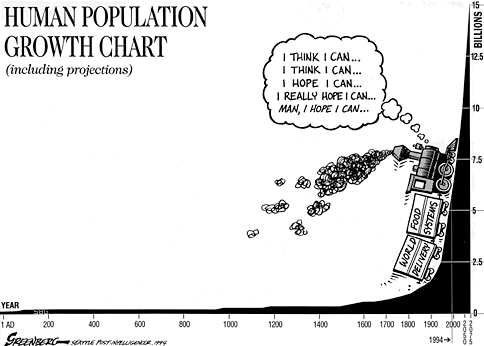# Mates y TIC - Maths and ICT

## Actividades de Matemáticas con TIC - Math Activities with ICT - - - (matesytic@gmail.com) Ricardo García Mesa

•## Piecewise, Exponential and Logarithmic functions

Posted by ricardogm on April 27th, 2016Hi

1. First of all, I’m going to show you how to draw piecewise functions. Pay attention.

2. Now you can repeat the exercises from class with geogebra. Send me one file for the piecewise functions and another one with the hyperbolas.

3.The teacher is going to show you an example of using Excel to calculate exponential growth. Do the same thing with this situation: A candy costs 0.05 € today, and the inflation is 4 % a year. Calculate the cost up to 1000 years.4. Last thing: When is the candy going to cost exactly 0.50 €? There are two ways to calculate it, the first one trial and error, and the second one is logarithms. Find out what logarithms have to do with this question. Send me the answer.¿What do you think about the question in this cartoon?Este artículo ha sido visitado  561  veces